# semiconductor physics,unit 5

13. Mar 2013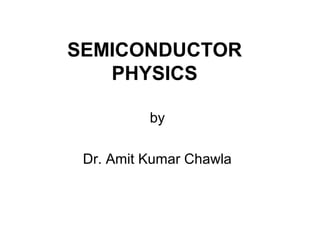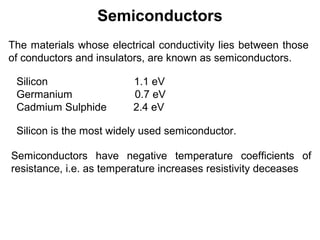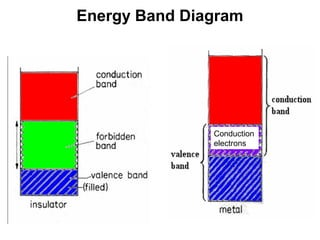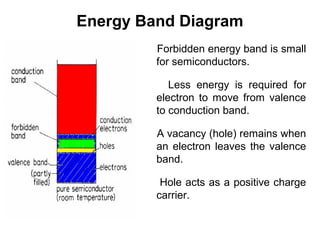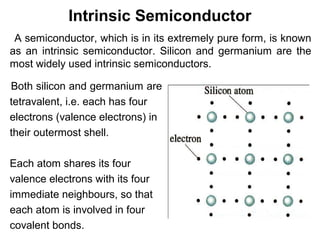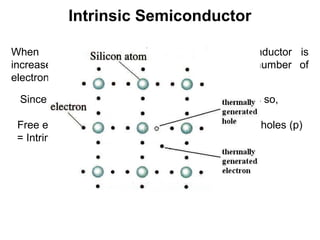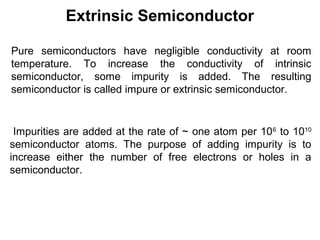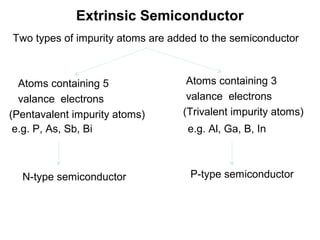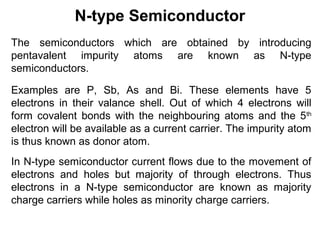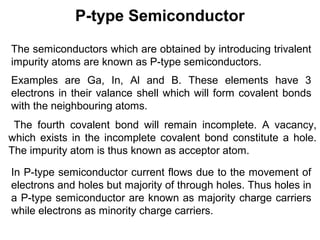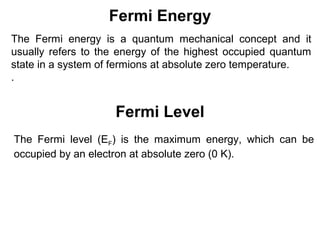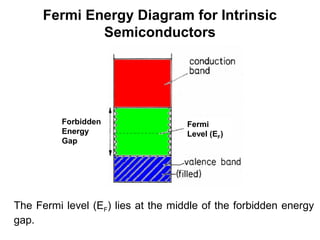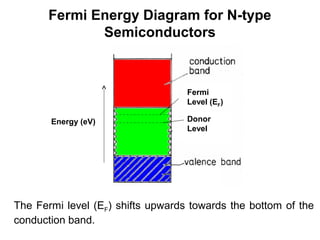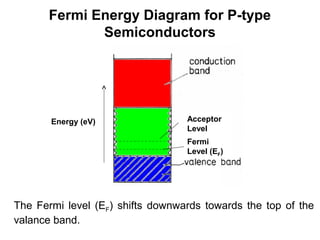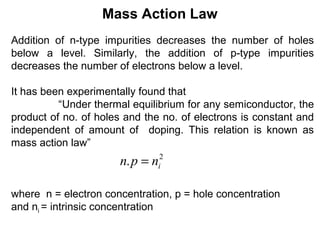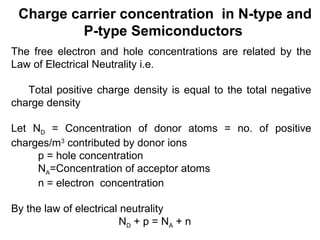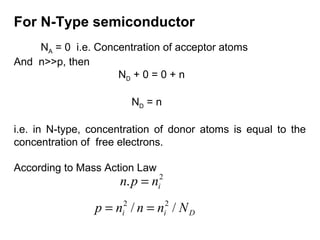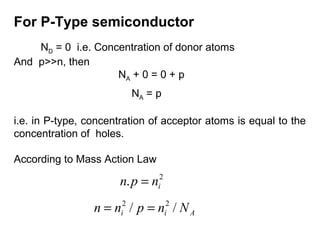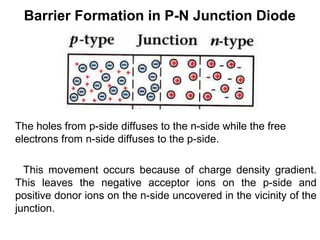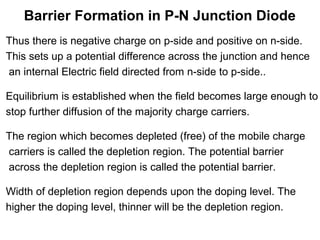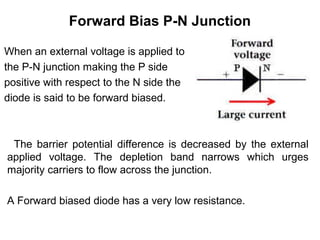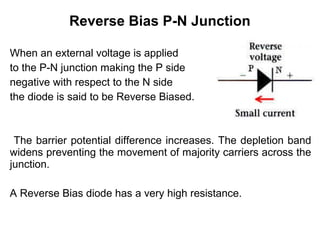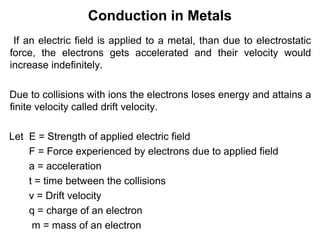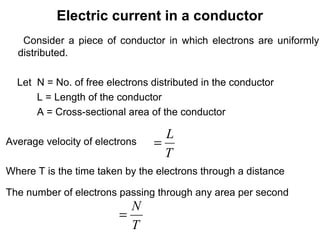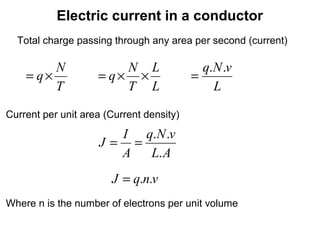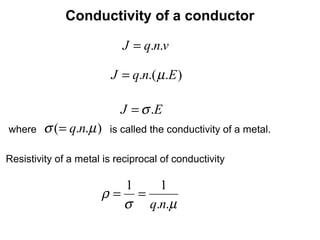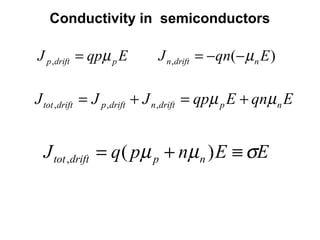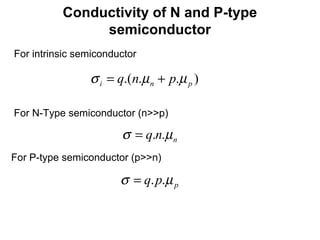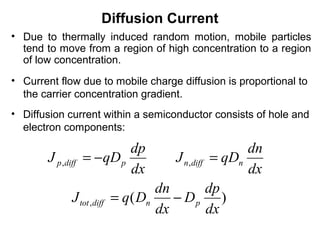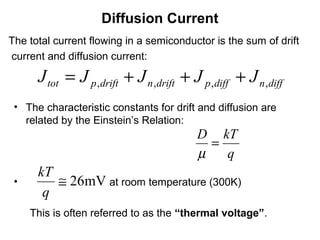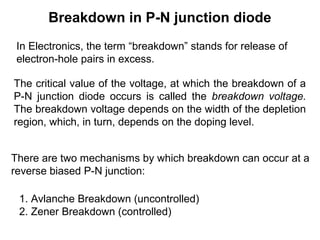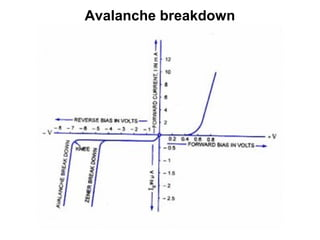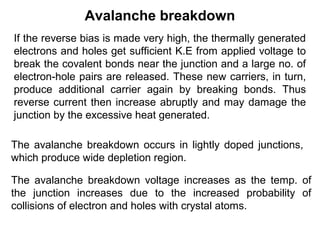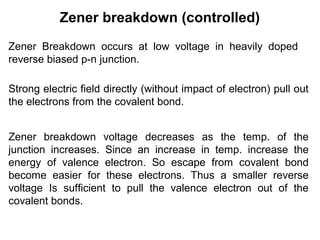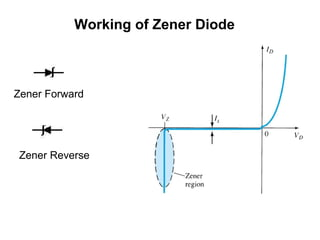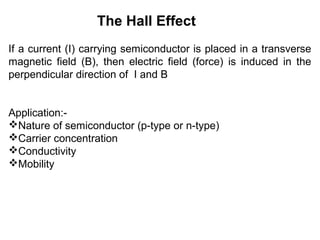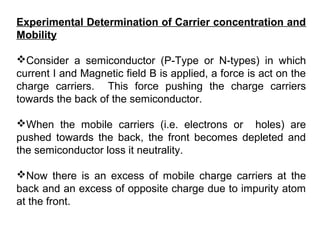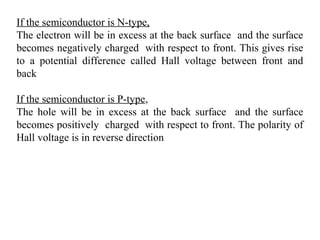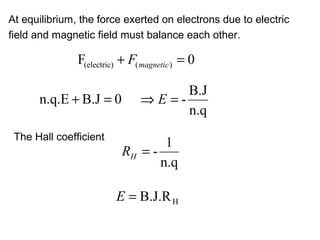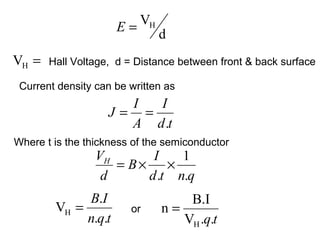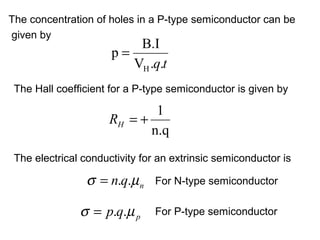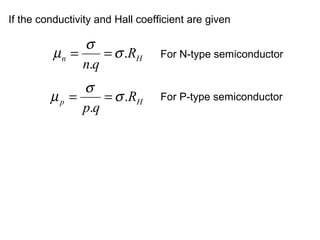1 von 42

### semiconductor physics,unit 5

• 1. SEMICONDUCTOR PHYSICS by Dr. Amit Kumar Chawla
• 2. Semiconductors The materials whose electrical conductivity lies between those of conductors and insulators, are known as semiconductors. Silicon 1.1 eV Germanium 0.7 eV Cadmium Sulphide 2.4 eV Silicon is the most widely used semiconductor. Semiconductors have negative temperature coefficients of resistance, i.e. as temperature increases resistivity deceases
• 3. Energy Band Diagram Conduction electrons
• 4. Energy Band Diagram Forbidden energy band is small for semiconductors. Less energy is required for electron to move from valence to conduction band. A vacancy (hole) remains when an electron leaves the valence band. Hole acts as a positive charge carrier.
• 5. Intrinsic Semiconductor A semiconductor, which is in its extremely pure form, is known as an intrinsic semiconductor. Silicon and germanium are the most widely used intrinsic semiconductors. Both silicon and germanium are tetravalent, i.e. each has four electrons (valence electrons) in their outermost shell. Each atom shares its four valence electrons with its four immediate neighbours, so that each atom is involved in four covalent bonds.
• 6. Intrinsic Semiconductor When the temperature of an intrinsic semiconductor is increased, beyond room temperature a large number of electron-hole pairs are generated. Since the electron and holes are generated in pairs so, Free electron concentration (n) = concentration of holes (p) = Intrinsic carrier concentration (ni)
• 7. Extrinsic Semiconductor Pure semiconductors have negligible conductivity at room temperature. To increase the conductivity of intrinsic semiconductor, some impurity is added. The resulting semiconductor is called impure or extrinsic semiconductor. Impurities are added at the rate of ~ one atom per 10 6 to 1010 semiconductor atoms. The purpose of adding impurity is to increase either the number of free electrons or holes in a semiconductor.
• 8. Extrinsic Semiconductor Two types of impurity atoms are added to the semiconductor Atoms containing 5 Atoms containing 3 valance electrons valance electrons (Pentavalent impurity atoms) (Trivalent impurity atoms) e.g. P, As, Sb, Bi e.g. Al, Ga, B, In N-type semiconductor P-type semiconductor
• 9. N-type Semiconductor The semiconductors which are obtained by introducing pentavalent impurity atoms are known as N-type semiconductors. Examples are P, Sb, As and Bi. These elements have 5 electrons in their valance shell. Out of which 4 electrons will form covalent bonds with the neighbouring atoms and the 5th electron will be available as a current carrier. The impurity atom is thus known as donor atom. In N-type semiconductor current flows due to the movement of electrons and holes but majority of through electrons. Thus electrons in a N-type semiconductor are known as majority charge carriers while holes as minority charge carriers.
• 10. P-type Semiconductor The semiconductors which are obtained by introducing trivalent impurity atoms are known as P-type semiconductors. Examples are Ga, In, Al and B. These elements have 3 electrons in their valance shell which will form covalent bonds with the neighbouring atoms. The fourth covalent bond will remain incomplete. A vacancy, which exists in the incomplete covalent bond constitute a hole. The impurity atom is thus known as acceptor atom. In P-type semiconductor current flows due to the movement of electrons and holes but majority of through holes. Thus holes in a P-type semiconductor are known as majority charge carriers while electrons as minority charge carriers.
• 11. Fermi Energy The Fermi energy is a quantum mechanical concept and it usually refers to the energy of the highest occupied quantum state in a system of fermions at absolute zero temperature. . Fermi Level The Fermi level (EF) is the maximum energy, which can be occupied by an electron at absolute zero (0 K).
• 12. Fermi Energy Diagram for Intrinsic Semiconductors Forbidden Fermi Energy Level (EF) Gap The Fermi level (EF) lies at the middle of the forbidden energy gap.
• 13. Fermi Energy Diagram for N-type Semiconductors Fermi Level (EF) Energy (eV) Donor Level Fermi Level (EF) The Fermi level (EF) shifts upwards towards the bottom of the conduction band.
• 14. Fermi Energy Diagram for P-type Semiconductors Energy (eV) Acceptor Level Fermi Level (EF) Fermi Level (EF) The Fermi level (EF) shifts downwards towards the top of the valance band.
• 15. Mass Action Law Addition of n-type impurities decreases the number of holes below a level. Similarly, the addition of p-type impurities decreases the number of electrons below a level. It has been experimentally found that “Under thermal equilibrium for any semiconductor, the product of no. of holes and the no. of electrons is constant and independent of amount of doping. This relation is known as mass action law” n. p = ni2 where n = electron concentration, p = hole concentration and ni = intrinsic concentration
• 16. Charge carrier concentration in N-type and P-type Semiconductors The free electron and hole concentrations are related by the Law of Electrical Neutrality i.e. Total positive charge density is equal to the total negative charge density Let ND = Concentration of donor atoms = no. of positive charges/m3 contributed by donor ions p = hole concentration NA=Concentration of acceptor atoms n = electron concentration By the law of electrical neutrality ND + p = NA + n
• 17. For N-Type semiconductor NA = 0 i.e. Concentration of acceptor atoms And n>>p, then ND + 0 = 0 + n ND = n i.e. in N-type, concentration of donor atoms is equal to the concentration of free electrons. According to Mass Action Law n. p = n 2 i p = n / n = n / ND 2 i 2 i
• 18. For P-Type semiconductor ND = 0 i.e. Concentration of donor atoms And p>>n, then NA + 0 = 0 + p NA = p i.e. in P-type, concentration of acceptor atoms is equal to the concentration of holes. According to Mass Action Law n. p = n 2 i n = n / p = n / NA 2 i 2 i
• 19. Barrier Formation in P-N Junction Diode The holes from p-side diffuses to the n-side while the free electrons from n-side diffuses to the p-side. This movement occurs because of charge density gradient. This leaves the negative acceptor ions on the p-side and positive donor ions on the n-side uncovered in the vicinity of the junction.
• 20. Barrier Formation in P-N Junction Diode Thus there is negative charge on p-side and positive on n-side. This sets up a potential difference across the junction and hence an internal Electric field directed from n-side to p-side.. Equilibrium is established when the field becomes large enough to stop further diffusion of the majority charge carriers. The region which becomes depleted (free) of the mobile charge carriers is called the depletion region. The potential barrier across the depletion region is called the potential barrier. Width of depletion region depends upon the doping level. The higher the doping level, thinner will be the depletion region.
• 21. Forward Bias P-N Junction When an external voltage is applied to the P-N junction making the P side positive with respect to the N side the diode is said to be forward biased. The barrier potential difference is decreased by the external applied voltage. The depletion band narrows which urges majority carriers to flow across the junction. A Forward biased diode has a very low resistance.
• 22. Reverse Bias P-N Junction When an external voltage is applied to the P-N junction making the P side negative with respect to the N side the diode is said to be Reverse Biased. The barrier potential difference increases. The depletion band widens preventing the movement of majority carriers across the junction. A Reverse Bias diode has a very high resistance.
• 23. Conduction in Metals If an electric field is applied to a metal, than due to electrostatic force, the electrons gets accelerated and their velocity would increase indefinitely. Due to collisions with ions the electrons loses energy and attains a finite velocity called drift velocity. Let E = Strength of applied electric field F = Force experienced by electrons due to applied field a = acceleration t = time between the collisions v = Drift velocity q = charge of an electron m = mass of an electron
• 24. Electric current in a conductor Consider a piece of conductor in which electrons are uniformly distributed. Let N = No. of free electrons distributed in the conductor L = Length of the conductor A = Cross-sectional area of the conductor L Average velocity of electrons = T Where T is the time taken by the electrons through a distance The number of electrons passing through any area per second N = T
• 25. Electric current in a conductor Total charge passing through any area per second (current) N N L q.N .v = q× = q× × = T T L L Current per unit area (Current density) I q.N .v J= = A L. A J = q.n.v Where n is the number of electrons per unit volume
• 26. Conductivity of a conductor J = q.n.v J = q.n.( µ.E ) J = σ .E where σ (= q.n.µ ) is called the conductivity of a metal. Resistivity of a metal is reciprocal of conductivity 1 1 ρ= = σ q.n.µ
• 27. Conductivity in semiconductors J p ,drift = qpµ p E J n ,drift = − qn(− µ n E ) J tot ,drift = J p ,drift + J n ,drift = qpµ p E + qnµ n E J tot ,drift = q ( pµ p + nµ n ) E ≡ σE
• 28. Conductivity of N and P-type semiconductor For intrinsic semiconductor σ i = q.(n.µ n + p.µ p ) For N-Type semiconductor (n>>p) σ = q.n.µ n For P-type semiconductor (p>>n) σ = q. p.µ p
• 29. Diffusion Current • Due to thermally induced random motion, mobile particles tend to move from a region of high concentration to a region of low concentration. • Current flow due to mobile charge diffusion is proportional to the carrier concentration gradient. • Diffusion current within a semiconductor consists of hole and electron components: dp dn J p ,diff = − qD p J n ,diff = qDn dx dx dn dp J tot ,diff = q ( Dn − Dp ) dx dx
• 30. Diffusion Current The total current flowing in a semiconductor is the sum of drift current and diffusion current: J tot = J p ,drift + J n ,drift + J p ,diff + J n ,diff • The characteristic constants for drift and diffusion are related by the Einstein’s Relation: D kT = µ q kT • ≅ 26mV at room temperature (300K) q This is often referred to as the “thermal voltage”.
• 31. Breakdown in P-N junction diode In Electronics, the term “breakdown” stands for release of electron-hole pairs in excess. The critical value of the voltage, at which the breakdown of a P-N junction diode occurs is called the breakdown voltage. The breakdown voltage depends on the width of the depletion region, which, in turn, depends on the doping level. There are two mechanisms by which breakdown can occur at a reverse biased P-N junction: 1. Avlanche Breakdown (uncontrolled) 2. Zener Breakdown (controlled)
• 33. Avalanche breakdown If the reverse bias is made very high, the thermally generated electrons and holes get sufficient K.E from applied voltage to break the covalent bonds near the junction and a large no. of electron-hole pairs are released. These new carriers, in turn, produce additional carrier again by breaking bonds. Thus reverse current then increase abruptly and may damage the junction by the excessive heat generated. The avalanche breakdown occurs in lightly doped junctions, which produce wide depletion region. The avalanche breakdown voltage increases as the temp. of the junction increases due to the increased probability of collisions of electron and holes with crystal atoms.
• 34. Zener breakdown (controlled) Zener Breakdown occurs at low voltage in heavily doped reverse biased p-n junction. Strong electric field directly (without impact of electron) pull out the electrons from the covalent bond. Zener breakdown voltage decreases as the temp. of the junction increases. Since an increase in temp. increase the energy of valence electron. So escape from covalent bond become easier for these electrons. Thus a smaller reverse voltage Is sufficient to pull the valence electron out of the covalent bonds.
• 35. Working of Zener Diode Zener Forward Zener Reverse
• 36. The Hall Effect If a current (I) carrying semiconductor is placed in a transverse magnetic field (B), then electric field (force) is induced in the perpendicular direction of I and B Application:- Nature of semiconductor (p-type or n-type) Carrier concentration Conductivity Mobility
• 37. Experimental Determination of Carrier concentration and Mobility Consider a semiconductor (P-Type or N-types) in which current I and Magnetic field B is applied, a force is act on the charge carriers. This force pushing the charge carriers towards the back of the semiconductor. When the mobile carriers (i.e. electrons or holes) are pushed towards the back, the front becomes depleted and the semiconductor loss it neutrality. Now there is an excess of mobile charge carriers at the back and an excess of opposite charge due to impurity atom at the front.
• 38. If the semiconductor is N-type, The electron will be in excess at the back surface and the surface becomes negatively charged with respect to front. This gives rise to a potential difference called Hall voltage between front and back If the semiconductor is P-type, The hole will be in excess at the back surface and the surface becomes positively charged with respect to front. The polarity of Hall voltage is in reverse direction
• 39. At equilibrium, the force exerted on electrons due to electric field and magnetic field must balance each other. F(electric) + F( magnetic ) = 0 B.J n.q.E + B.J = 0 ⇒E =- n.q The Hall coefficient 1 RH = - n.q E = B.J.R H
• 40. VH E= d VH = Hall Voltage, d = Distance between front & back surface Current density can be written as I I J= = A d .t Where t is the thickness of the semiconductor VH I 1 = B× × d d .t n.q B.I B.I VH = or n= n.q.t VH .q.t
• 41. The concentration of holes in a P-type semiconductor can be given by B.I p= VH .q.t The Hall coefficient for a P-type semiconductor is given by 1 RH = + n.q The electrical conductivity for an extrinsic semiconductor is σ = n.q.µ n For N-type semiconductor σ = p.q.µ p For P-type semiconductor
• 42. If the conductivity and Hall coefficient are given σ µn = = σ .RH For N-type semiconductor n.q σ µp = = σ .RH For P-type semiconductor p.q

1. Vbc cgvg